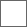• 24回复贴，共1

### 黑体辐射与热体辐射的差别

2019-11-14 07:17 广告

β=3α，或，α=β/3E=Vσ=Vρ(μ+δ(1-ρb/ρ)+εB+u^2)=W(μ+δ(1-ρb/ρ)+εB+u^2)

Q=Ed=NR

P=ρu^2
PV=Q=NR，R=rT，其中，r理想气体常数
PV=NrT=Q
PV/R=Q/R=N
PV/NR=Q/NR=1，即
PVo/R=1E=U+K

H=S+R

E=NH，U=NS，K=NR

γ=(V-ΔV)/V=1-ΔV/V

β=3α，或，α=β/3
γ＝1-βΔR
E=U+K=NH=N(S+R)
ΔE=ΔU+ΔK=Δ(NH)

ΔE=ΔQ

ΔQ=ΔQi+ΔQν+ΔQe

ΔW=ΔWi+ΔWνΔQ=ΔQi+ΔQν+ΔQr#### 扫二维码下载贴吧客户端